High Power Laser Science and Engineering, 2023, 11 (4): 04000e49, Published Online: Jul. 10, 2023

### Phase imaging of irradiated foils at the OMEGA EP facility using phase-stepping X-ray Talbot–Lau deflectometry

2 Université de Bordeaux-CNRS-CEA, Centre Lasers Intenses et Applications (CELIA), UMR 5107, Talence, France
3 European XFEL GmbH, Schenefeld, Germany
4 Laboratory for Laser Energetics, Rochester, New York, USA
5 CEA-DAM, DIF, Arpajon, France
6 University of Michigan, Ann Arbor, Michigan, USA
7 Los Alamos National Laboratory, Los Alamos, New Mexico, USA
8 Lawrence Livermore National Laboratory, Livermore, California, USA
9 Helmholtz-Zentrum Dresden-Rossendorf, Dresden, Germany
10 Technische Universität Dresden, Dresden, Germany
11 Institute of Physics of the ASCR, Prague, Czech Republic
12 CEA-CESTA, CS 60001, Le Barp Cedex, France
13 Blackett Laboratory, Imperial College London, London, UK
14 Department of Astrophysics and Astronomy, The Johns Hopkins University, Baltimore, Maryland, USA
15 Center for Energy Research, University of California San Diego, San Diego, California, USA
Abstract
Diagnosing the evolution of laser-generated high energy density (HED) systems is fundamental to develop a correct understanding of the behavior of matter under extreme conditions. Talbot–Lau interferometry constitutes a promising tool, since it permits simultaneous single-shot X-ray radiography and phase-contrast imaging of dense plasmas. We present the results of an experiment at OMEGA EP that aims to probe the ablation front of a laser-irradiated foil using a Talbot–Lau X-ray interferometer. A polystyrene (CH) foil was irradiated by a laser of 133 J, 1 ns and probed with 8 keV laser-produced backlighter radiation from Cu foils driven by a short-pulse laser (153 J, 11 ps). The ablation front interferograms were processed in combination with a set of reference images obtained ex situ using phase-stepping. We managed to obtain attenuation and phase-shift images of a laser-irradiated foil for electron densities above ${10}^{22}\;{\mathrm{cm}}^{-3}$ . These results showcase the capabilities of Talbot–Lau X-ray diagnostic methods to diagnose HED laser-generated plasmas through high-resolution imaging.

## 1 Introduction

Interferometry methods are a very powerful tool for diagnosing plasmas, as they can provide very valuable information about the plasma electron and ion density in a simple manner. However, current diagnostic methods mostly rely on visible radiation and, thus, high energy density (HED) plasma probing is difficult since these plasmas are mostly opaque to visible wavelengths. Considering this, Talbot–Lau interferometry is a promising approach to diagnosing HED plasmas as it extends interferometry methods to the X-ray regime.

Talbot–Lau interferometry relies on two different phenomena: the Talbot effect and the Lau effect. The Talbot effect exhibits itself in the fact that when a coherent wave is diffracted by a periodic grating, an exact replica of the grating is observed at a distance ${z}_{\mathrm{T}}$ (called the Talbot length) from the grating. On top of that, at fractional distances of this length, a similar image, with different periodicity, is also produced[4,5]. The distances of interest are denoted as ${d}_m=\frac{m}{4}{z}_{\mathrm{T}}$ , where $m$ is an integer number called the Talbot order (even for transmission gratings, and odd for phase gratings). For X-ray radiation and $\unicode{x3bc} \mathrm{m}$ -periodic gratings, these distances are of the order of approximately $10{-}100\;\mathrm{cm}$ , which makes Talbot–Lau interferometers easy to operate and implement.

Since most laboratory X-ray sources are incoherent per se, as is the case for laser-produced X-ray sources, they cannot be used directly to generate an interference pattern. However, a series of smaller and locally coherent sources can be generated through the Lau effect by placing an additional diffraction grating between the source and the interferometer. Under the right conditions, this so-called source grating can generate an interference pattern.

These two effects are combined in a Talbot–Lau interferometer as follows. After the backlighter, the first diffraction grating (the source grating) is placed in front of the interferometer to generate locally coherent sources from the probing radiation. The object to be probed is often placed next, so that it imprints a phase pattern on the incoming radiation. This is then diffracted by a second grating called the beamsplitter or phase grating. The diffraction pattern that is generated is finally filtered by a third grating called the analyzer, placed at a distance ${d}_m$ from the beamsplitter. This last grating turns the small phase changes into larger intensity changes that can be projected onto a detector. In the case where there is no object to be probed, an exact replica of the beamsplitter would be obtained at ${d}_m$ (as per the Talbot and Lau effects). However, when an object is introduced in the interferometer, it modifies the coherence of the wave, and thus the Talbot pattern. These deviations from the ideal case can then be used to infer information about the properties of the object. A schematic drawing of a Talbot–Lau interferometer (corresponding to the experimental setup described in Section 2) is shown in Figure 1(a).#### Fig. 1. (a) Schematic drawing of the experimental setup. The figure shows the different elements of the interferometer together with the backlighter target, the plasma target and the corresponding laser beams. In this figure,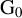corresponds to the source grating,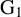is the beamsplitter andis the analyzer grating described in the text. The dot-dashed line across all elements corresponds to the optical axis of the interferometer. Note that the distances indicated between the different elements are not to scale. (b) Schematic drawing describing how the phase-stepping procedure works. The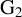grating is displaced perpendicular to the instrument axis and grating bars, scanning over one full grating period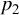() after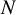steps. In this schematic, only four steps in a period are shown and the rotation angle of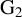with respect to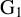has been exaggerated for clarity (in our experimental setup, this angle was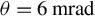).

Talbot–Lau interferometry has been widely used in the field of medical sciences, since it permits imaging softer tissue than traditional X-ray radiography while keeping a high resolution. In these cases it is common to use the method of phase-stepping, which consists of taking several sequential images for laterally incremental positions of the analyzer grating, spanning one period, as shown schematically in Figure 1(b). The set of images can be used to reconstruct high-resolution phase and transmission images. Consequently, eliminating any non-data-related defects in the images (such as grating imperfections, dead pixels in the detector or similar) is enabled by the method, as only the periodic features are kept. In addition, the position of the analyzer grating that maximizes image contrast can be found by following the intensity curve[9,10], which can be used to obtain refraction-enhanced X-ray images.

In recent years, with the aim of imaging dense plasmas, there have been several efforts to adapt Talbot–Lau interferometry to high-power laser facilities such as PALS, the Multi-Terawatt (MTW) facility and OMEGA EP, as well as proof-of-concept experiments at lower-energy high-repetition rate lasers. These experiments have permitted a thorough study of the optimal requirements for X-ray backlighting, while also providing useful data for analysis tool development. In such experiments, phase-stepping methods are not commonly used, owing to the limited amount of data that can be usually obtained at high-power laser facilities, which impedes taking several images in the same conditions for different positions of the analyzer grating. Furthermore, driver and backlighter laser beams are often close enough to the source grating to cause grating ablation. Therefore, instead of phase-stepping, a deflectometry configuration is used, where the analyzer grating is rotated a small angle $\theta$ with respect to the beamsplitter (also shown schematically in Figure 1(b)). This generates a Moiré pattern on the image that introduces an additional periodicity, larger than that of the individual gratings ( $\sim p/\theta$ , where $p$ is the original period of the analyzer). Over this new periodicity, it is possible to measure perturbations caused by the object that is being probed.

It is in this context that we present the results from a recent experiment at the OMEGA EP laser facility[30,31], where we used Talbot–Lau X-ray interferometry to image the ablation front of a laser-generated plasma, combining Moiré deflectometry and phase-stepping techniques. While Moiré deflectometry was used to image the expanding plasma, reference images (without the plasma) were taken by applying phase-stepping to the same Moiré interferometer configuration. In this case, the reference images can be taken ex situ using a continuous laboratory X-ray source instead of the laser-produced X-ray backlighter source. Note that, as mentioned above, reference image acquisition is a significant limitation in a high-power laser system. Thus, we take advantage of the additional information provided by phase-stepping, while being able to use the Moiré deflectometry technique. We obtained, for the first time, X-ray transmission and phase-shift information of a laser-produced compression wave through a solid. This set of data can provide useful information about the ion and electron density distribution of the plasma.

This paper is structured as follows. In Section 2, a detailed description of the experimental configuration and setup is given, describing both the interferometer and the laser configuration as well as the HED experiment performed. In this section, we also describe how the interferometry reference images were obtained. Section 3 describes the method for analyzing the resulting interferograms, both for the object and the set of reference images. The results from the experiment are shown and discussed in Section 4. Finally, Section 5 presents a summary of the results as well as plans for future improvements to the diagnostic technique.

## 2 Experimental setup

The experiment was conducted at the OMEGA Extended Performance (EP) facility[30,31], using an identical setup to that described by Valdivia et al.. A $125\;\unicode{x3bc} \mathrm{m}$ thick polystyrene (CH) foil, with mass density $\rho =1.05\;\mathrm{g}/{\mathrm{cm}}^3$ , was driven with a 133 J, 1 ns laser pulse at $3\omega$ ( $\lambda =351\;\mathrm{nm}$ ), focused to an eighth-order supergaussian focal spot, with 95% of the energy contained within a $365\;\unicode{x3bc} \mathrm{m}$ radius, corresponding to a laser intensity on target of $3.45\times {10}^{13}\;\mathrm{W}\;{\mathrm{cm}}^{-2}$ . The generated plasma was then probed at ${t=500\;\mathrm{ps}}$ (where $t=0$ corresponds to the start of the $3\omega$ drive) using a $20\;\unicode{x3bc} \mathrm{m}$ -thick Cu backlighter foil. The backlighting radiation was generated using the short-pulse capability at OMEGA EP, with a 153 J, 11 ps pulse at $1\omega$ ( $\lambda =1053\;\mathrm{nm}$ ). A zinc von Hamos spectrometer (ZVH) and a dual channel highly oriented pyrolytic graphite spectrometer (DCHOPG) were fielded to characterize the X-ray spectrum of the backlighter.

The Talbot–Lau interferometer was designed to work with 8 keV illumination and thus uses the K-shell emission from the copper backlighter target ( $\lambda =0.154$ nm). The instrument was composed of three gratings, hereafter referred to as $\mathrm{G}_0$ , $\mathrm{G}_1$ and $\mathrm{G}_2$ for simplicity, which operate as the source, beamsplitter and analyzer grating, respectively.

A montage of the interferometer is shown in Figure 1(a). It corresponds to the $m=7$ Talbot order. This configuration yields a Talbot magnification ( ${d}_{\mathrm{G}_0\mathrm{G}_2}/{d}_\mathrm{G_0\mathrm{G}_1}$ ) of ${M}_{\mathrm{T}}\sim 6$ and object magnification ( ${d}_{\mathrm{G}_0\mathrm{G}_2}/{d}_{\mathrm{G}_0 \mathrm{Ob}}$ ) of ${M}_{\mathrm{Ob}}\sim 41$ .

In Figure 1(a), the backlighter target (Cu foil) is shown on the left, and the plasma (CH foil) target is shown in the middle. The two laser beams are also shown along with the position and periodicities of the $\mathrm{G}_0$ , $\mathrm{G}_1$ and $\mathrm{G}_2$ gratings with respect to the target and backlighter. A $20\;\unicode{x3bc} \mathrm{m}$ -thick Cu filter was mounted in front of $\mathrm{G}_0$ to filter the high-energy emission from the backlighter. In addition, a $15\;\unicode{x3bc} \mathrm{m}$ -thick Al filter and a $25\;\unicode{x3bc} \mathrm{m}$ -thick Cu filter were mounted in front of the beamsplitter ( $\mathrm{G}_1$ grating) and the detector, respectively, to filter the low-energy contribution from the target self-emission (the combined transmission of these last two filters was $<1\%$ for photon energies below 5 keV).

The $\mathrm{G}_2$ grating was rotated with respect to the $\mathrm{G}_1$ grating so that their grating bars had a small angle (sin $\theta \approx \theta$ ) with respect to each other, thus enabling Moiré deflectometry. In our case, $\mathrm{G}_2$ was rotated $6\;\mathrm{mrad}$ with respect to $\mathrm{G}_1$ to generate a Moiré periodicity of approximately $50\;\unicode{x3bc} \mathrm{m}$ on target, corresponding to approximately $150\;\mathrm{pixels}$ on the detector, to maximize the signal and facilitate the detection of the fringe structure in a single shot.#### Fig. 2. (a) Example of ex situ reference images recorded for phase-stepping. The Moiré fringes are oriented horizontally. The red line shows the average normalized fringe profile, corresponding to a contrast of approximately 20%. (b) Normalized intensity phase curve corresponding to all phase-stepping reference images. The so-called phase-stepping contrast (contrast of the phase-stepping features) is 20%.

While most of the backlighter emission corresponds to Cu-K $\unicode{x3b1}$ radiation, we observed a significant contribution from the He $\unicode{x3b1}$ and K $\unicode{x3b2}$ lines (which are not removed by the $\mathrm{G}_0$ filter) in addition to a minor bremsstrahlung contribution. This non-monochromaticity has been shown to reduce the Moiré contrast by a factor of 1.9–2.2. It is worth mentioning that this contrast reduction is observed in the absence of self-emitting plasma.

A series of 15 different reference images were obtained outside of the experimental chamber in order to maximize data collection during the experiments. A medical-grade rotating Cu anode X-ray tube with a focal spot of $15\;\unicode{x3bc} \mathrm{m}$ at full width at half maximum (FWHM) was used to reproduce the 8 keV emission from the backlighter (more details on this source can be found in Ref. , along with its X-ray spectrum). Note that in previous works we observed no significant effects when using different Cu X-ray sources for the reference and data images, since most of the backlighter structure is removed by the data analysis.

The reference images were obtained using the phase-stepping technique, where the analyzer grating was shifted perpendicularly to the interferometer line-of-sight over one period ( $12\;\unicode{x3bc} \mathrm{m}$ ) in approximately $1.3\;\unicode{x3bc} \mathrm{m}$ steps. Since these reference images were obtained upon finalization of the alignment procedure of the interferometer and prior to shots, the position and orientation of the gratings are identical to those used to collect the data and, thus, reference phase maps can be obtained.

An example of these reference images is presented in Figure 2(a), clearly showing the interference Moiré fringes. The red line in the image indicates the normalized intensity profile (averaged), which shows that a $20\%$ contrast was obtained for each image recorded in the phase-stepping procedure. It can be seen that the fringes in the image bend slightly around $x=50\;\unicode{x3bc} \mathrm{m}$ . This non-uniformity in the Moiré fringe pattern is caused by source grating defects[22,35]. As the non-uniform region is consistently localized in images, this defect vanishes when analyzing the periodicity of the Moiré pattern by means of a Fourier analysis and, thus, its effect can be neglected. In any case, for further confidence, this region was removed in the later analysis.

Figure 2(b) presents the interferometer phase curve, which is obtained from the phase-stepping procedure. Here, the normalized average intensity within a central $10\;\unicode{x3bc} \mathrm{m}$ -wide horizontal strip (shaded blue region in Figure 2(a)) is determined for each phase-stepped Moiré image. Each intensity value shown corresponds to a single position of the analyzer grating in the phase-stepping process. The error bars correspond to the standard error of the signal in that region. Interferometer contrast of approximately 20% was measured from this phase curve. This value is similar to the contrast calculated using fringe intensity values for each individual reference Moiré image in the phase-stepping image set. Note that, while the periodicity of the curve presented in Figure 2(b) is independent of the region that is considered, the normalized intensity values are different for each pixel position.

## 3 Analysis of the interferograms

The Moiré images obtained both for the object and reference have two main components, namely the underlying image and the periodic contribution that arises from the interferometry itself. In the case of the reference image, since phase-stepping was used, there is an additional periodic component (corresponding to the shifting of the analyzer grating) that must be taken into account. For this reason, the obtained interferograms can be written as follows:

\begin{align}{I}_{\mathrm{obj}}\left(\mathbf{r}\right)={A}_{\mathrm{obj}}\left(\mathbf{r}\right)+{B}_{\mathrm{obj}}\left(\mathbf{r}\right){e}^{i\left[{\mathbf{k}}_{\mathrm{f}}\cdot \mathbf{r}+{\phi}_{\mathrm{obj}}\left(\mathbf{r}\right)\right]},\end{align}

and

\begin{align}{I}_{\mathrm{ref}}\left(\mathbf{r},n\right)={A}_{\mathrm{ref}}\left(\mathbf{r}\right)+{B}_{\mathrm{ref}}\left(\mathbf{r}\right){e}^{i\left[{\mathbf{k}}_{\mathrm{f}}\cdot \mathbf{r}+{\phi}_{\mathrm{ref}}\left(\mathbf{r}\right)+\frac{2\pi n}{T}\right]},\end{align}

where $I$ is the intensity of the signal. The sub-indices ‘obj’ and ‘ref’ refer to the object and reference images, respectively, ${\mathbf{k}}_{\mathrm{f}}$ corresponds to the periodicity of the fringes and $\mathbf{r}=\left(x,y\right)$ indicates the position of a given pixel. Here, $A$ and $B$ are real functions corresponding to the intensity of the underlying signal and the periodic contribution, respectively. In the case of the reference image, the index $n$ corresponds to the $n$ th reference image taken in the phase-stepping process, while $T$ indicates the number of steps that span a full period of the grating (from Figure 2(b) it can be seen that in this case $T=9$ ).

In the equations above, the contribution $A$ is equivalent to a regular X-ray radiograph. This is due to the fact that X-rays refracted within the plasma will interact with those diffracted by the gratings, affecting the periodic part of the interferometry image, either via the $B$ or the $\phi$ functions. Therefore, an equivalent attenuation image can be obtained from the following:

\begin{align}\mathrm{Attenuation}\left(\mathbf{r}\right)=\frac{A_{\mathrm{obj}}\left(\mathbf{r}\right)}{A_{\mathrm{ref}}\left(\mathbf{r}\right)}.\end{align}

The functions $B$ and $\phi$ contain the information about X-rays refracted within the plasma, which can be extracted in two different ways. It can be easily seen that $B/A$ corresponds to the relative intensity of the interferometry features on the images, that is, the contrast of the fringes, or visibility. The relative change in the visibility of the fringes introduced by the object is denoted as darkfield[4,36].

On the other hand, $\phi$ indicates deviations from the perfect periodicity (which would be given only by the term $\exp \left(i{\mathbf{k}}_{\mathrm{f}}\cdot \mathbf{r}\right)$ ) and, therefore, it is a metric of how much the fringes have shifted from their non-perturbed position. In the ideal case, ${\phi}_{\mathrm{obj}}$ would be an absolute measurement, without the need for a reference, since ${\phi}_{\mathrm{ref}}=0$ , as the reference image would be perfectly periodical. However, this is not usually the case, owing to multiple causes such as the finite size of the image, the presence of noise or the shape of the backlighter illumination pattern, grating structure and/or imperfections. For this reason, the phase shift introduced by the object is calculated in practice, as follows:

\begin{align}\phi \left(\mathbf{r}\right)={\phi}_{\mathrm{obj}}\left(\mathbf{r}\right)-{\phi}_{\mathrm{ref}}\left(\mathbf{r}\right).\end{align}

Separating the $A$ and $\phi$ components of the interferograms is therefore necessary to obtain any physical information from both the transmission of the plasma and the phase shift it introduces. Below, we detail how this process works for both the cases with and without phase-stepping.

## 3.1 Object image: no phase-stepping

To extract the different components of the object interferogram, the easiest way is to work in Fourier space and express the obtained image as a function of its frequency components. In this case,

\begin{align}{I}_{\mathrm{obj}}\left(\mathbf{r}\right)={\int}_{-\infty}^{\infty }F\left(\mathbf{k}\right){e}^{i\mathbf{k}\cdot \mathbf{r}}\mathrm{d}\mathbf{k},\end{align}

where $F\left(\mathbf{k}\right)$ is the Fourier transform of ${I}_{\mathrm{obj}}$ . From Equation (1), it is clear that the function $F$ is heavily weighted towards the frequency of the interference fringes, ${\mathbf{k}}_{\mathrm{f}}$ . We can then define two functions ${F}_1$ and ${F}_2$ such that $F={F}_1+{F}_2$ , as follows:

\begin{align}{F}_1\left(\mathbf{k}\right)=\left\{\begin{array}{ll}F\left(\mathbf{k}\right),& \left|\mathbf{k}-{\mathbf{k}}_{\mathrm{f}}\right|>\delta, \\ {}0,& \left|\mathbf{k}-{\mathbf{k}}_{\mathrm{f}}\right|<\delta, \end{array}\right.\end{align}

\begin{align}{F}_2\left(\mathbf{k}\right)=F\left(\mathbf{k}\right)-{F}_1\left(\mathbf{k}\right),\end{align}

where $\delta$ defines a small interval around the natural frequency of the fringes ${\mathbf{k}}_{\mathrm{f}}$ . With these definitions, ${F}_1$ corresponds to the Fourier transform of the original image ${I}_{\mathrm{obj}}$ , once the interferometry fringes (and their possible shifts) have been removed, whereas ${F}_2$ exclusively contains information about the fringes in ${I}_{\mathrm{obj}}$ . Therefore, by inverting the Fourier transform, we obtain the following:

\begin{align}{A}_{\mathrm{obj}}\left(\mathbf{r}\right)={\mathrm{\mathcal{F}}}^{-1}\left({F}_1\right),\end{align}

and

\begin{align}{B}_{\mathrm{obj}}\left(\mathbf{r}\right){e}^{i\left[{\mathbf{k}}_{\mathrm{f}}\cdot \mathbf{r}+{\phi}_{\mathrm{obj}}\left(\mathbf{r}\right)\right]}={\mathrm{\mathcal{F}}}^{-1}\left({F}_2\right),\end{align}

where the symbol ${\mathrm{\mathcal{F}}}^{-1}$ denotes the inverse Fourier transform. The $B$ and $\phi$ components are easily separated by taking the natural logarithm of Equation (9), yielding the following:

\begin{align}\log \left[{B}_{\mathrm{obj}}\left(\mathbf{r}\right)\right]=\operatorname{Re}\left\{\log \left[{\mathrm{\mathcal{F}}}^{-1}\left({F}_2\right)\right]\right\},\end{align}

and

\begin{align}{\mathbf{k}}_{\mathrm{f}}\cdot \mathbf{r}+{\phi}_{\mathrm{obj}}\left(\mathbf{r}\right)=\operatorname{Im}\left\{\log \left[{\mathrm{\mathcal{F}}}^{-1}\left({F}_2\right)\right]\right\}.\end{align}

## 3.2 Reference image: phase-stepping

For the case of the reference images, the process is similar. However, we take advantage of the additional periodicity introduced by the phase-stepping (see Figure 2(b)). For every point $\mathbf{r}$ , the set of images $I\left(\mathbf{r},n\right)$ is described in terms of a Fourier series as follows:

\begin{align}{I}_{\mathrm{ref}}\left(\mathbf{r},n\right)=\sum \limits_{j=-\infty}^{\infty }{Q}_j\left(\mathbf{r}\right){e}^{i\frac{2\pi j}{T}n},\end{align}

where $n$ and $T$ have the same meaning as in Equation (2), and the factors ${Q}_j\left(\mathbf{r}\right)$ are the weights of each term in the Fourier series. Comparing Equations (2) and (12), the following is clear:

\begin{align}{Q}_0\left(\mathbf{r}\right)={A}_{\mathrm{ref}}\left(\mathbf{r}\right),\end{align}

and

\begin{align}{Q}_1\left(\mathbf{r}\right)={B}_{\mathrm{ref}}\left(\mathbf{r}\right){e}^{i\left[{\mathbf{k}}_{\mathrm{f}}\cdot \mathbf{r}+{\phi}_{\mathrm{ref}}\left(\mathbf{r}\right)\right]}.\end{align}

By taking the real and imaginary parts of the natural logarithm of ${Q}_1\left(\mathbf{r}\right)$ , the terms ${B}_{\mathrm{ref}}\left(\mathbf{r}\right)$ and ${\mathbf{k}}_{\mathrm{f}}\cdot \mathbf{r}+{\phi}_{\mathrm{ref}}\left(\mathbf{r}\right)$ can be obtained identically as in the case with no phase-stepping.

Once the functions $A$ , $B$ and $\phi$ have been obtained for both the object and reference images, it is possible to calculate the attenuation and phase-shift maps from Equations (3) and (4) (note that the terms ${\mathbf{k}}_{\mathrm{f}}\cdot \mathbf{r}$ cancel out when carrying out the subtraction in Equation (4)).

## 4 Results

Figure 3 shows the Moiré interferogram obtained in the experiment. The signal-to-noise ratio (SNR) in the data was approximately 1.7, which lowered the Moiré fringe contrast further beyond the limitations imposed by the non-monochromaticity of the backlighter emission (as mentioned in Section 2). The central dark region corresponds to the target that is being ablated, whereas the interferometry fringes can be seen on both sides of it. The target was irradiated from the left-hand side. Similar to Figure 2(a), the fringe profile is shown in red. A contrast of $5\%$ was measured in the region outside the plasma target, while inside the target the contrast decreases ( $3\%$ ) as a result of reduced X-ray transmission. This lower Moiré fringe contrast is due to increased X-ray emission above 8 keV.#### Fig. 3. Interferometry image of the ablated plasma. The dark vertical feature aroundcorresponds to the CH foil. Note that the direction of the phase-change detection coincides with the Moiré fringes, which are oriented horizontally in our experiment. The region indicated with the red-dashed square corresponds to the field of view of the reference images and, therefore, to the region that was analyzed. The region within the white-dotted square corresponds to the region of consideration, after removing the edges to avoid the Gibbs phenomenon and possible grating imperfections. Similar to Figure 2(a), the fringe profile inside the target is shown on the left-hand side of the image, corresponding to approximately 3% fringe contrast (the contrast outside the target is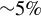). Note that the contrast plot has been scaled to improve the view.

Since the detector used for Talbot–Lau rail X-ray alignment and calibration has a smaller chip size than that used in the experiment, the field of view of the reference images was smaller than that of the object image. To obtain a direct comparison, the field of view of the images recorded in the experiment was reduced to the region shown in the figure with a red-dashed square.

The postprocessing module of the Talbot Interferometry Analysis (TIA) code, named the Talbot Numerical Tool (TNT), was used to separate the different Fourier components of both the data and reference images, following the procedure described in the previous section. This tool has been developed to automatically detect the peaks corresponding to the periodicity of the fringes in the Fourier spectra of noisy images, which was fundamental to detect and analyze the low-contrast fringes in the data presented.

In order to benchmark the obtained results, we ran 2D simulations of the plasma ablation with the magneto-hydrodynamics (MHD) code FLASH, using the experimental parameters. The simulations were run using adaptative mesh refinement (AMR) with a maximum spatial resolution of $1\;\unicode{x3bc} \mathrm{m}$ . The effects of self-generated B-fields (through the Biermann battery effect), although negligible, were included in the simulations using ideal MHD models. The target was initialized at solid density, with an ionization of $0.1$ , submerged in a fully ionized He background with ion density ${n}_\mathrm{i}={10}^{13}\;{\mathrm{cm}}^{-3}$ . The equations of state and opacity tables for the target and the surrounding low-density gas were obtained with the code PropacEOS. Simulations were run with a Courant–Friedrichs–Lewy number (CFL) of $0.3$ . Since solid targets in FLASH are modeled as high-density gases, some numerical diffusion in the rear part of the target is possible. While this effect is negligible for early probing times, such as that considered in this work (0.5 ns), to avoid numerical noise, we froze the rear part of the target in the simulation to prevent it from expanding before the shock reaches it.

A summary of the results is presented in Figure 4, where Figures 4(b) and 4(c) correspond to the attenuation and phase-shift curves, respectively, integrated over the region of interest. The shaded regions in each plot mark the original position and size of the CH target, after accounting for the spatial resolution of the instrument. In order to avoid edge effects or the Gibbs phenomenon from the Fourier analysis, the data in Figure 4 correspond to 75% of the effective field of view (white square in Figure 3). Figure 4(a) shows a close-up of the interferometry image in the region of interest for reference. In addition, guiding lines (vertical red-dashed lines) have been added in order to indicate the region of the target that has been shocked by the laser drive.

In Figure 4(b), it can be seen that while the transmission of radiation through the target is constant in the unshocked material, this is not the case for the shocked region. In said region (between the red guiding lines), the transmission is lower as the material density is increased. Around $475\;\unicode{x3bc} \mathrm{m}$ , the slope in the transmission curve corresponds to the target edge convoluted with the spatial resolution of the instrument ( $20\;\unicode{x3bc} \mathrm{m}$ ). In addition, at the rear edge of the target, it can be seen that the transmission rises significantly above one. This is a common effect in X-ray radiography, known as refraction enhancement[41,42], in which the contrast of an object with sharp edges and features is enhanced and these features appear brighter in X-ray imaging. This effect is commonly taken advantage of for detecting irregularities in target fabrication for cases where smoothness of the targets is critical.#### Fig. 4. (a) Cropped region from the interferometry data corresponding to the field of view of the reference images (red square in Figure 3) after removing the image edges to avoid the Gibbs phenomenon from the Fourier analysis. The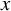and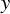coordinates correspond to the white-dotted square in Figure 3. (b), (c) Transmission and phase-shift data line-outs, integrated over the region shown in (a). The shaded regions in all images correspond to the original position of the target convoluted with the spatial resolution of the instrument. In (c) the brown dotted line corresponds to the phase shift obtained without applying phase-stepping techniques, while the black dashed line corresponds to the phase shift obtained from the FLASH simulations, by taking the integrated electron density gradient. The vertical dashed lines across all figures correspond to the expanding plasma.

At the time of consideration (0.5 ns after the start of the laser pulse), the shocked region of the target has not yet heated up and ionized. Therefore, it is possible to use the tabulated values of the mass absorption coefficient for cold polystyrene to obtain an estimate of the mass density of the target from the transmission data. By assuming uniform density across the laser spot ( $730\;\unicode{x3bc} \mathrm{m}$ in diameter), and using a two-densities model (one value for the shocked material and one for the unshocked target), the density of the shocked region (between the red lines) is estimated to be ${2.1\pm 0.5\;\mathrm{g}/{\mathrm{cm}}^3}$ , which indicates a compression of about a factor of two with respect to the initial target density of $1.05\;\mathrm{g}/{\mathrm{cm}}^3$ . The error bars in this measurement arise from the 95% confidence interval obtained by performing a statistical t-test on the transmission data from the compressed region with respect to the data in the uncompressed region. This is in good agreement with the value predicted by the FLASH simulations $\left(2.3\;\mathrm{g}/{\mathrm{cm}}^3\right)$ . The obtained density profile is shown as the yellow line in Figure 4(a).

In Figure 4(c), we have included the phase shift obtained by using the phase-stepping analysis described above, indicated with a green solid line, together with the equivalent result obtained without phase-stepping (i.e., using a single, best-correlated reference image), marked with a brown dotted line. Notably, this highlights the benefits of the phase-stepping technique, without which no phase structure can be seen in the data. The rise in the absolute value of the phase shift observed at both edges of the target is caused by the gradient in electron density from the CH target to the vacuum. The error region corresponds to a $5\%$ fringe shift.

In order to compare the obtained phase shifts with the simulations, we note that the phase shift is directly related to the electron density of the plasma, as follows:

\begin{align}\frac{\partial }{\partial x}\left(\int {n}_{\mathrm{e}} \mathrm{d}z\right)=\frac{\phi \left(\mathbf{r}\right)}{\lambda^2{r}_{\mathrm{e}}}\cdot \frac{p_0}{d_0},\end{align}

where ${n}_{\mathrm{e}}$ is the electron density, $z$ is the direction of the line-of-sight, $x$ is the same as used in Figure 4, $\lambda$ is the wavelength of the radiation probing the plasma, ${r}_{\mathrm{e}}$ is the classical electron radius and ${p}_0$ and ${d}_0$ are the periodicity of the source grating ( $\mathrm{G}_0$ ) and its distance to the target, respectively[15,47,48].

The phase shift obtained from the simulations is shown in Figure 4(c) as the black dashed line. Good agreement is found between the simulations and the measured values, especially for the front of the target ( $x\sim 310\;\unicode{x3bc} \mathrm{m}$ ), where the fringes are most visible. It is worth noting that in this region, the electron density is two to four times higher than the critical density for $4\omega$ Thomson scattering ( ${n}_{\mathrm{c}}=1.6\times {10}^{22}\;{\mathrm{cm}}^{-3}$ ). This shows the capabilities and potential advantages of Talbot–Lau interferometry to diagnose dense plasmas further than traditional methods allow. Considering that the spatial resolution of the system was $20\;\unicode{x3bc} \mathrm{m}$ (which can be further improved in future iterations) and the SNR was $1.7$ , most of the features in the phase curve are blurred and only the peaks corresponding to the target edges are discernible. This is clear in the FLASH curve, where both edge features at 310 and at $475\;\unicode{x3bc} \mathrm{m}$ look very similar (the change in the electron density gradient caused by the target compression has been almost washed out by the instrument resolution).

The second y-axis in Figure 4(c) indicates the refraction angle of the X-rays for different regions of the target. This angle $\alpha$ is directly related to the phase shift, as follows:

\begin{align}\alpha =\frac{\phi \left(\mathbf{r}\right)}{2\pi}\cdot \frac{p_0}{d_0}=F\left(\mathbf{r}\right)\cdot {W}_{\mathrm{eff}},\end{align}

where $F\left(\mathbf{r}\right)=\phi \left(\mathbf{r}\right)/2\pi$ is the phase shift measured in units of fringes, and ${W}_{\mathrm{eff}}={p}_0/{d}_0$ is the angular sensitivity of the instrument. From Equation (16), it follows that ${W}_{\mathrm{eff}}$ is the refraction angle that results in one fringe shift on the deflectometry image. In this configuration, ${W}_{\mathrm{eff}}=150\;\unicode{x3bc} \mathrm{rad}$ (as obtained from the values in Figure 1(a)).

Note that, while the critical density for 8 keV photon energy is $4.6\times {10}^{28}\;{\mathrm{cm}}^{-3}$ , the limitations for this measurement are given by Equation (15). Although the upper limit of fringe shift measurements depends on the interferometer contrast, spatial resolution and SNR, it has been shown that fringe shifts up to $F\sim 5$ can be measured with this technique, which imposes an upper limit in the gradient on the integrated electron density (not the electron density per se) of $7\times {10}^{25}\;{\mathrm{cm}}^{-3}$ in order for the phase shift to be measurable. By assuming uniform density over the diameter of the laser spot ( $730\;\unicode{x3bc} \mathrm{m}$ ) and that gradients are detectable over $20\;\unicode{x3bc} \mathrm{m}$ (the spatial resolution of the instrument), the maximum electron density that can be probed with this setup can be estimated to be ${n}_{\mathrm{e}}^{\mathrm{max}}=2\times {10}^{24}\;{\mathrm{cm}}^{-3}$ . In contrast, the minimum density values that can be detected ( $F\sim 0.05$ ) are ${n}_{\mathrm{e}}^{\mathrm{min}}=2\times {10}^{22}\;{\mathrm{cm}}^{-3}$ , close to the values expected in this experiment.

## 5 Conclusions and future work

We have presented the first X-ray interferometry image of an ablating HED plasma obtained at a high-power laser facility, probed by a Talbot–Lau X-ray interferometer. From this image, both phase-contrast radiography (attenuation) and phase-shift data of the ablating plasma have been retrieved.

By using ex situ phase-stepping, we were able to obtain phase-stepped reference images for our analysis, without loss of beamtime. This allowed us to obtain high-quality phase-shift data despite marginal photon statistics, reflected by the low SNR of the interferometry signal ( $<2$ ) and poor Moiré fringe contrast ( $3\%{-}5\%$ ). The phase-stepping process has proved crucial in order to extract any phase-shift information, since no structure is observed if single-image analysis is used.

The obtained data show different features from the ablated plasma and the target edge. In particular, an absolute measurement of the phase shift from the ablated plasma has been obtained, finding good agreement with the predictions from hydrodynamic simulations, which indicates that the electron density is above the range that can be probed with optical means. This proves that the Talbot–Lau interferometry technique can be used in HED experiments to characterize and map the hydrodynamic evolution and behavior of laser-driven plasmas well above ${10}^{22}\;{\mathrm{cm}}^{-3}$ , thus enabling characterization in regions unavailable with current diagnostics.

The data obtained with this technique can provide meaningful insights into the physics of the ablation zone in laser-generated plasmas (a requirement to benchmark current theoretical models), as it allows direct probing into the dense ablated regions. In addition, this experimental platform and diagnostic can be used to study the process of species separation in plasmas with more than one element (such as CH), which is necessary to discriminate among diffusion models.

Future work includes further developments to the OMEGA EP Talbot–Lau X-ray Deflectometer (EP-TXD) diagnostic to enhance signal quality and fringe contrast by improving spatial resolution and X-ray backlighter spectra. Backlighter target geometry and orientation with respect to the incident laser will be explored to improve system spatial resolution and overall signal quality in combination with optimization of the laser parameters. The addition of a laterally graded multilayer mirror to the OMEGA EP-TXD will ensure monochromaticity of the X-ray backlighter radiation by removing contributions from higher energy lines (such as the He $\unicode{x3b1}$ or the K $\unicode{x3b2}$ lines) and hot electron re-circulation, among others, which have shown to be detrimental to TXD diagnostic accuracy. In addition, both TIA and its postprocessing module the TNT will be further developed to work with additional hydrodynamic codes and deliver real-time information, providing valuable insight to inform experimental campaigns.

References

 D.Stutman and M.Finkenthal, Rev. Sci. Instrum.82, 113508 (2011).

 H. F.Talbot, London, Edinburgh, Dublin Philos. Mag. J. Sci.9, 401 (1836).

 E.Lau, Ann. Phys.437, 417 (1948).

 V.Bouffetier, L.Ceurvorst, M.Valdivia, F.Dorchies, S.Hulin, T.Goudal, D.Stutman, and A.Casner, Appl. Opt.59, 8380 (2020).

 G.Pérez-Callejo, V.Bouffetier, L.Ceurvorst, T.Goudal, M.Valdivia, D.Stutman, and A.Casner, Phys. Plasmas29, 043901 (2022).

 A.Momose, W.Yashiro, H.Maikusa, and Y.Takeda, Opt. Express17, 12540 (2009).

 F.Pfeiffer, O.Bunk, C.David, M.Bech, G.Le Duc, A.Bravin, and P.Cloetens, Phys. Med. Biol.52, 6923 (2007).

 T.Weitkamp, C.David, O.Bunk, J.Bruder, P.Cloetens, and F.Pfeiffer, Eur. J. Radiol.68, S13 (2008).

 Y.Surrel, Appl. Opt.32, 3598 (1993).

 Y.Surrel, Appl. Opt.35, 51 (1996).

 M. P.Valdivia, G.Perez-Callejo, V.Bouffetier, G. W.Collins, C.Stoeckl, T.Filkins, C.Mileham, M.Romanofsky, I. A.Begishev, W.Theobald, S. R.Klein, M. K.Schneider, F. N.Beg, A.Casner, and D.Stutman, Rev. Sci. Instrum.93, 115102 (2022).

 J.Nejdl, M.Kozlová, T.Mocek, and B.Rus, Phys. Plasmas17, 122705 (2010).

 M.Valdivia, D.Stutman, C.Stoeckl, W.Theobald, C.Mileham, I.Begishev, J.Bromage, and S.Regan, Rev. Sci. Instrum.87, 023505 (2016).

 M.Valdivia, D.Stutman, C.Stoeckl, C.Mileham, J.Zou, S.Muller, K.Kaiser, C.Sorce, P.Keiter, J.Fein, M.Trantham, R. P.Drake, and S. P.Regan, Rev. Sci. Instrum.91, 023511 (2020).

 M. P.Valdivia, F.Veloso, D.Stutman, C.Stoeckl, C.Mileham, I. A.Begishev, W.Theobald, M.Vescovi, W.Useche, S. P.Regan, B.Albertazzi, G.Rigon, P.Mabey, T.Michel, S. A.Pikuz, M.Koenig, and A.Casner, Rev. Sci. Instrum.89, 10G127 (2018).

 J.Krasinski, D. F.Heller, and O.Kafri, Appl. Opt.24, 3032 (1985).

 A.Momose, S.Kawamoto, I.Koyama, Y.Hamaishi, K.Takai, and Y.Suzuki, Jpn. J. Appl. Phys.42, L866 (2003).

 B.Moosman, V.Bystritskii, C.Boswell, and F.Wessel, Rev. Sci. Instrum.67, 170 (1996).

 J.Ruiz-Camacho, F.Beg, and P.Lee, J. Phys. D: Appl. Phys.40, 2026 (2007).

 J.Valenzuela, E.Wyndham, H.Chuaqui, D.Cortes, M.Favre, and H.Bhuyan, J. Appl. Phys.111, 103301 (2012).

 M.Valdivia, D.Stutman, and M.Finkenthal, J. Appl. Phys.114, 163302 (2013).

 M.Valdivia, D.Stutman, and M.Finkenthal, Rev. Sci. Instrum.85, 073702 (2014).

 M.Valdivia, D.Stutman, and M.Finkenthal, Appl. Opt.54, 2577 (2015).

 D.Stutman, M. P.Valdivia, and M.Finkenthal, Appl. Opt.54, 5956 (2015).

 J.Valenzuela, E.Wyndham, and M.Favre, Phys. Plasmas22, 083501 (2015).

 S.Bachche, M.Nonoguchi, K.Kato, M.Kageyama, T.Koike, M.Kuribayashi, and A.Momose, Sci. Rep.7, 6711 (2017).

 M.Seifert, M.Gallersdörfer, V.Ludwig, M.Schuster, F.Horn, G.Pelzer, J.Rieger, T.Michel, and G.Anton, J. Imaging4, 62 (2018).

 S.Balovsiak, S.Novikov, I.Fodchuk, and I.Yaremchuk, Metallophys. Adv. Technol.41, 389 (2019).

 H.Lee, D.Jeon, H.Lim, H.Cho, M.Park, and W.Youn, J. Opt.23, 105605 (2021).

 L.Waxer, D.Maywar, J.Kelly, T.Kessler, B.Kruschwitz, S.Loucks, R.McCrory, D.Meyerhofer, S.Morse, C.Stoeckl, and J.Zuegel, Opt. Photonics News16, 30 (2005).

 J.Kelly, L.Waxer, V.Bagnoud, I.Begishev, J.Bromage, B.Kruschwitz, T.Kessler, S.Loucks, D.Maywar, R.McCrory, D.Meyerhofer, S.Morse, J.Oliver, A.Rigatti, A.Schmid, C.Stoeckl, S.Dalton, L.Folnsbee, M.Guardalben, R.Jungquist, J.Puth, M.ShoupIII, D.Weiner, and J.Zuegel, J. Phys. IV133, 75 (2006).

 O.Kafri, Opt. Lett.5, 555 (1980).

 O.Kafri and A.Livnat, Appl. Opt.20, 3098 (1981).

 J.Stricker and O.Kafri, AIAA J.20, 820 (1982).

 M. P.Valdivia, D.Stutman, C.Stoeckl, C.Mileham, I. A.Begishev, J.Bromage, and S. P.Regan, Appl. Opt.57, 138 (2018).

 F.Pfeiffer, M.Bech, O.Bunk, P.Kraft, E. F.Eikenberry, C.Brönnimann, C.Grünzweig, and C.David, Nat. Mater.7, 134 (2008).

 B.Fryxell, K.Olson, P.Ricker, F.Timmes, M.Zingale, D.Lamb, P.MacNeice, R.Rosner, J.Truran, and H.Tufo, Astrophys. J. Suppl. Ser.131, 273 (2000).

 A.Dubey, K.Antypas, M. K.Ganapathy, L. B.Reid, K.Riley, D.Sheeler, A.Siegel, and K.Weide, Parallel Comput.35, 512 (2009).

 P.Tzeferacos, M.Fatenejad, N.Flocke, C.Graziani, G.Gregori, D.Lamb, D.Lee, J.Meinecke, A.Scopatz, and K.Weide, High Energy Density Phys.17, 24 (2015).

 J.MacFarlane, I.Golovkin, and P.Woodruff, J.Quant. Spectrosc. Radiat. Transfer99, 381 (2006).

 Y.Ping, O.Landen, D.Hicks, J.Koch, R.Wallace, C.Sorce, B.Hammel, and G.Collins, J. Instrum.6, P09004 (2011).

 J. A.Koch, O. L.Landen, L. J.Suter, and L. P.Masse, J. Opt. Soc. Am. A30, 1460 (2013).

 B.Kozioziemski, J.Koch, A.Barty, H.MartzJr, W.-K.Lee, and K.Fezzaa, J. Appl. Phys.97, 063103 (2005).

 J. A.Koch, O. L.Landen, B. J.Kozioziemski, N.Izumi, E. L.Dewald, J. D.Salmonson, and B. A.Hammel, J. Appl. Phys.105, 113112 (2009).

 J. A.Koch, O. L.Landen, L. J.Suter, L. P.Masse, D. S.Clark, J. S.Ross, A. J.Mackinnon, N. B.Meezan, C. A.Thomas, and Y.Ping, Appl. Opt.52, 3538 (2013).

 A.Momose, W.Yashiro, Y.Takeda, Y.Suzuki, and T.Hattori, Jpn. J. Appl. Phys.45, 5254 (2006).

 M.Born and E.Wolf, Principles of Optics: Electromagnetic Theory of Propagation, Interference and Diffraction of Light (Elsevier, Amsterdam, 2013).

 A.Momose, Jpn. J. Appl. Phys.44, 6355 (2005).

G. Pérez-Callejo, V. Bouffetier, L. Ceurvorst, T. Goudal, S. R. Klein, D. Svyatskiy, M. Holec, P. Perez-Martin, K. Falk, A. Casner, T. E. Weber, G. Kagan, M. P. Valdivia. Phase imaging of irradiated foils at the OMEGA EP facility using phase-stepping X-ray Talbot–Lau deflectometry[J]. High Power Laser Science and Engineering, 2023, 11(4): 04000e49.引用该论文： TXT   |   EndNote

### 相关论文